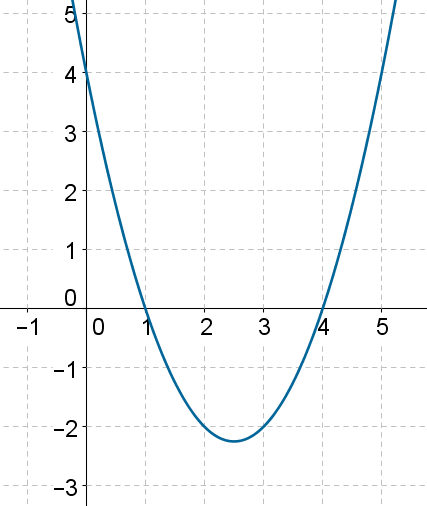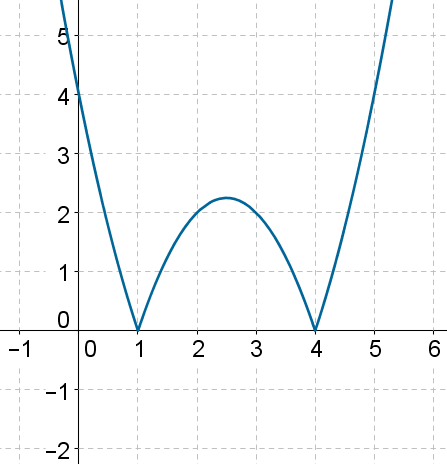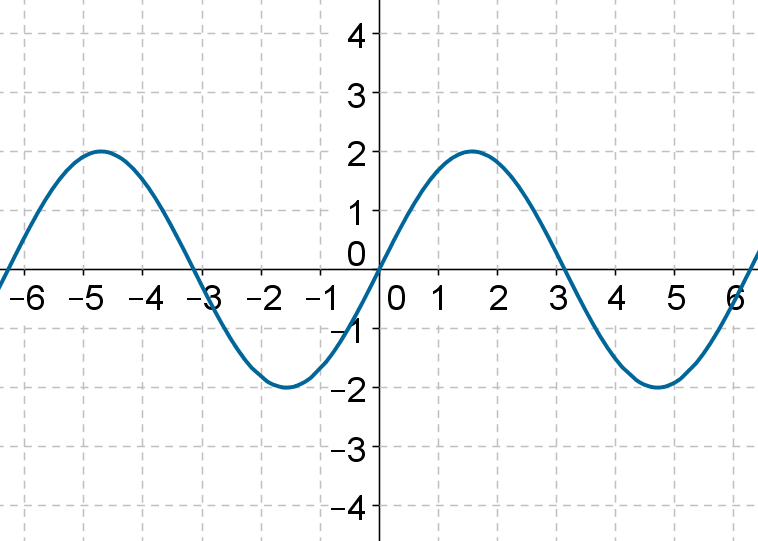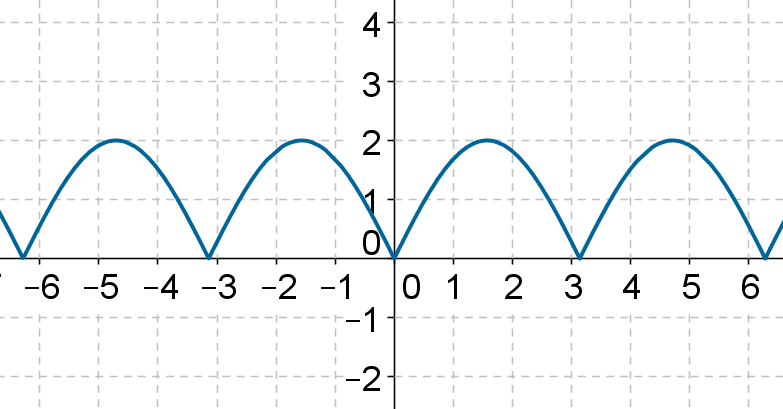# Applying modulus

Go back to  'Functions'

The following figure shows the graph of the function $$f\left( x \right) = {x^2} - 5x+ 4$$:Using this graph, can we plot the graph of $$y = g\left( x \right) = \left|{f\left( x \right)} \right|$$? The answer is simple: wherever f is positive, we leave the curve untouched; wherever f is negative, we reflect that part in the x-axis, because the effect of the modulus operation is to give us the positive magnitude. In this case, the graph of $$y = \left| {f\left( x \right)} \right|$$ will be as follows:Thus, the part of the curve below the x-axis in the original graph gets reflect in the x-axis.

Let’s see another example of this transformation. The following is the graph of $$y= f\left( x \right)$$, where f is some arbitrary function:And, the following is the graph of $$y = \left| {f\left( x \right)} \right|$$:Functions
Functions
grade 10 | Questions Set 2
Functions
Functions
grade 10 | Questions Set 1